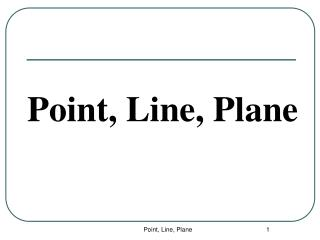DownloadDownload PresentationPoint, Line, Plane

# Point, Line, Plane

Télécharger la présentation## Point, Line, Plane

- - - - - - - - - - - - - - - - - - - - - - - - - - - E N D - - - - - - - - - - - - - - - - - - - - - - - - - - -
##### Presentation Transcript

1. Point, Line, Plane Point, Line, Plane

2. Geometry Terms • Undefined terms: words that do not have a formal definition but there is agreement about what they mean. • Defined terms: Terms that can be described using known words • Postulate or Axiom: Rule that is accepted without proof. • Theorem: Rule that can be proved. Point, Line, Plane

3. Points • Points do not have actual size. • How to Sketch: Using dots • How to label: Use capital letters Never name two points with the same letter (in the same sketch). A B A C Point, Line, Plane

4. Lines • Lines extend indefinitely and have no thickness or width. • How to sketch : using arrows at both ends. • How to name: 2 ways (1) small script letter – line n (2) any two points on the line - • Never name a line using three points - n A B C Point, Line, Plane

5. Collinear Points • Collinear points are points that lie on the same line. (The line does not have to be visible.) • A point lies on the line if the coordinates of the point satisfy the equation of the line. Ex: To find if A (1, 0) is collinear with the points on the line y = -3x + 3. Substitute x = 1 and y = 0 in the equation. 0 = -3 (1) + 3 0 = -3 + 3 0 = 0 The point A satisfies the equation, therefore the point is collinear with the points on the line. A B C Collinear C A B Non collinear Lesson 1-1 Point, Line, Plane

6. Planes • A plane is a flat surface that extends indefinitely in all directions. • How to sketch: Use a parallelogram (four sided figure) • How to name: 2 ways (1) Capital script letter – Plane M (2) Any 3 non collinear points in the plane - Plane: ABC/ ACB / BAC / BCA / CAB / CBA A M B C Horizontal Plane Vertical Plane Other Lesson 1-1 Point, Line, Plane

7. Different planes in a figure: A B Plane ABCD Plane EFGH Plane BCGF Plane ADHE Plane ABFE Plane CDHG Etc. D C E F H G Lesson 1-1 Point, Line, Plane

8. Other planes in the same figure: Any three non collinear points determine a plane! Plane AFGD Plane ACGE Plane ACH Plane AGF Plane BDG Etc. Lesson 1-1 Point, Line, Plane

9. Coplanar Objects Coplanar objects (points, lines, etc.) are objects that lie on the same plane. The plane does not have to be visible. Are the following points coplanar? A, B, C ? Yes A, B, C, F ? No H, G, F, E ? Yes E, H, C, B ? Yes A, G, F ? Yes C, B, F, H ? No Lesson 1-1 Point, Line, Plane

10. Intersection of Figures The intersection of two figures is the set of points that are common in both figures. The intersection of two lines is a point. m Line m and line n intersect at point P. P n Continued……. Lesson 1-1 Point, Line, Plane

11. 3 Possibilities of Intersection of a Line and a Plane (1) Line passes through plane – intersection is a point. (2) Line lies on the plane - intersection is a line. (3) Line is parallel to the plane - no common points. Lesson 1-1 Point, Line, Plane

12. Intersection of Two Planes is a Line. B P A R Plane P and Plane R intersect at the line Lesson 1-1 Point, Line, Plane

13. RA : RA and all points Y such that A is between R and Y. ( the symbol RA is read as “ray RA” ) Ray Definition: How to sketch: How to name: Lesson 1-2: Segments and Rays

14. Opposite Rays Definition: If A is between X and Y, AX and AY are opposite rays. ( Opposite rays must have the same “endpoint” ) opposite rays not opposite rays Lesson 1-2: Segments and Rays

15. Segment Part of a line that consists of two points called the endpoints and all points between them. Definition: How to sketch: How to name: AB (without a symbol) means the length of the segment or the distance between points A and B. Lesson 1-2: Segments and Rays

16. 12 AC + CB = AB x + 2x = 12 3x = 12 x = 4 The Segment Addition Postulate Postulate: If C is between A and B, then AC + CB = AB. If AC = x , CB = 2x and AB = 12, then, find x, AC and CB. Example: 2x x Step 1: Draw a figure Step 2: Label fig. with given info. Step 3: Write an equation x = 4 AC = 4 CB = 8 Step 4: Solve and find all the answers Lesson 1-2: Segments and Rays

17. Homework • Pg. 5 # 1 • Pg 6 # 17, 18, 20 • Pg 12 # 8, 10, 12 • Pg 13 #21 to 26, 29 Lesson 1-2: Formulas